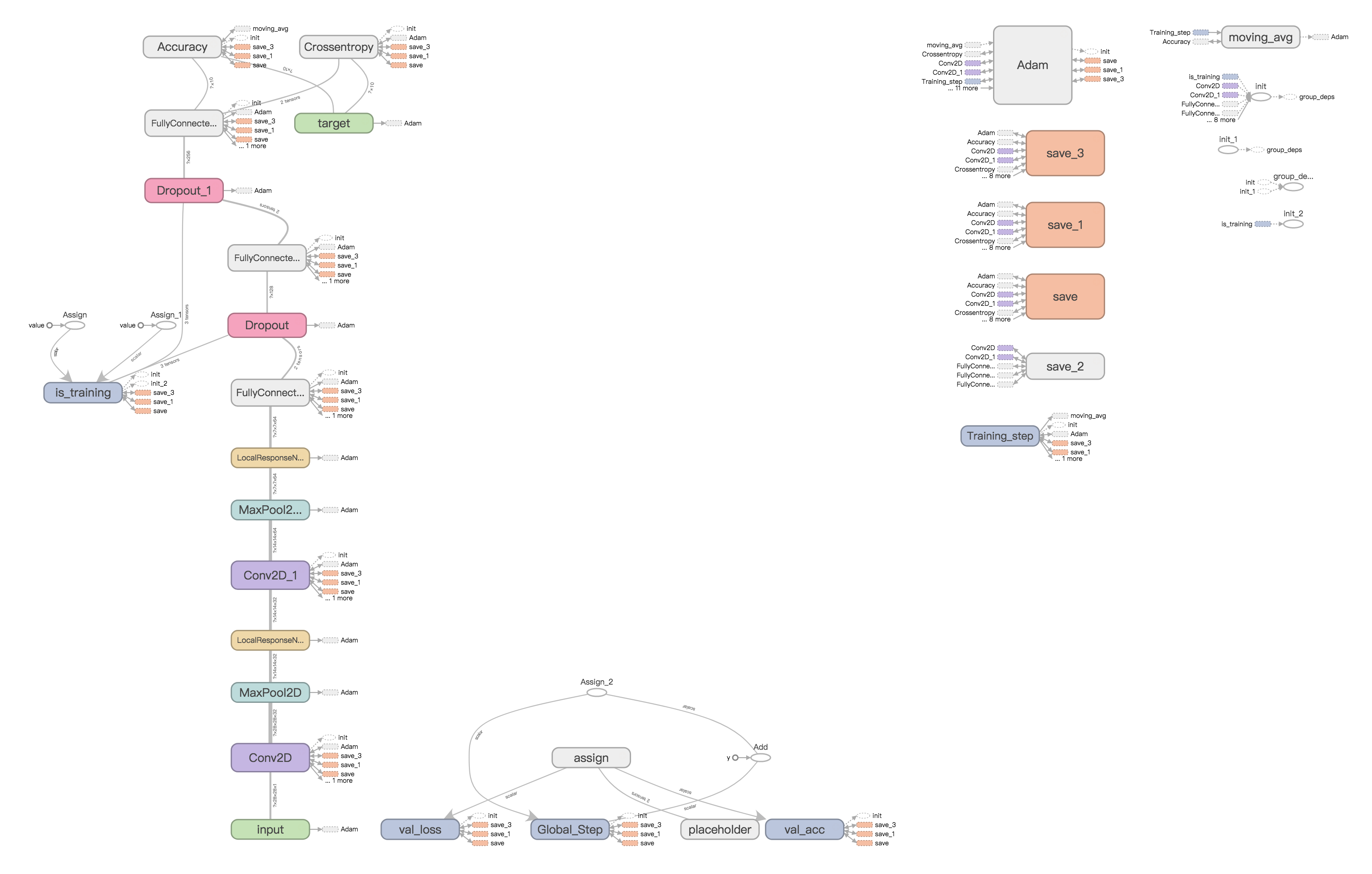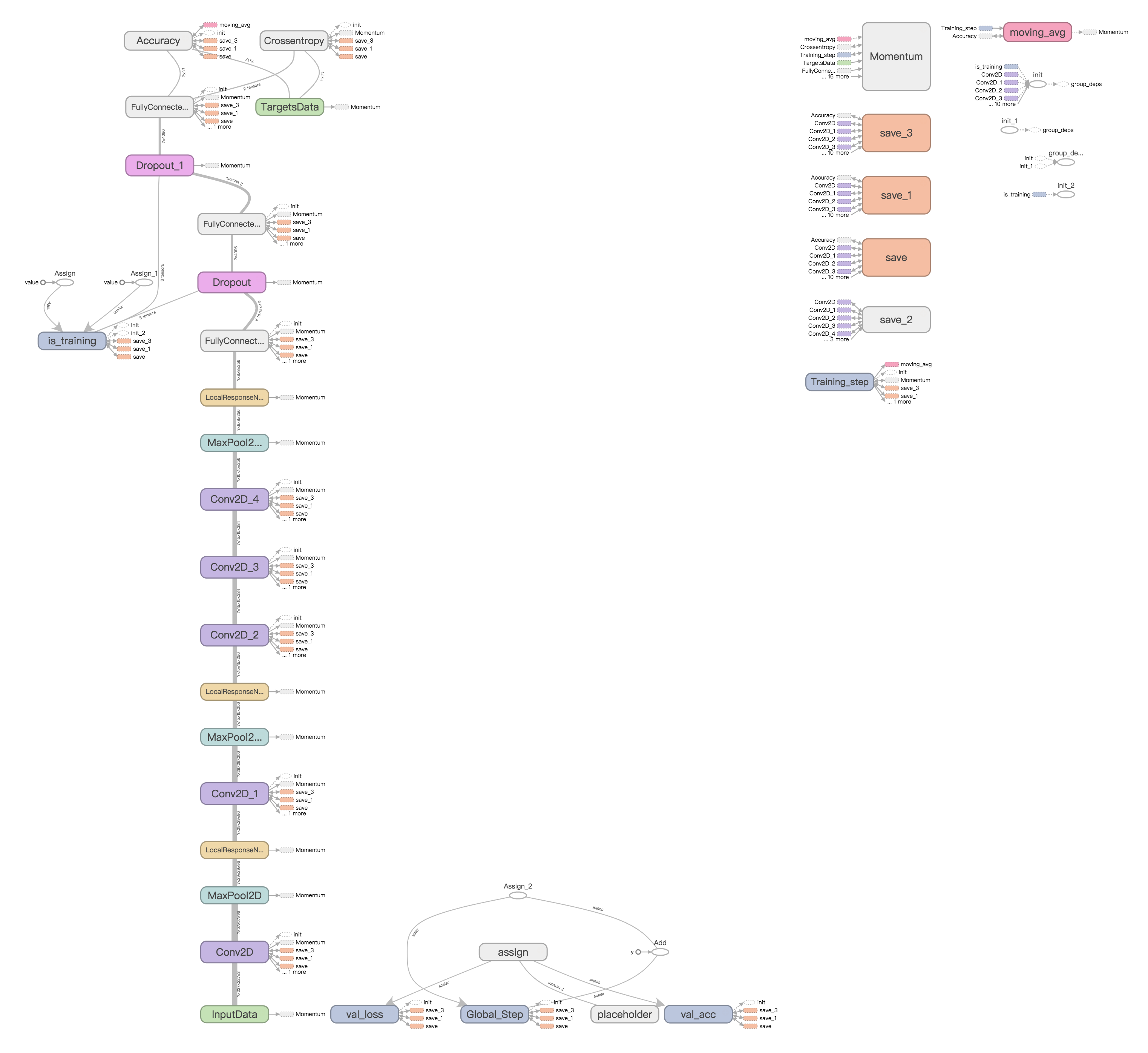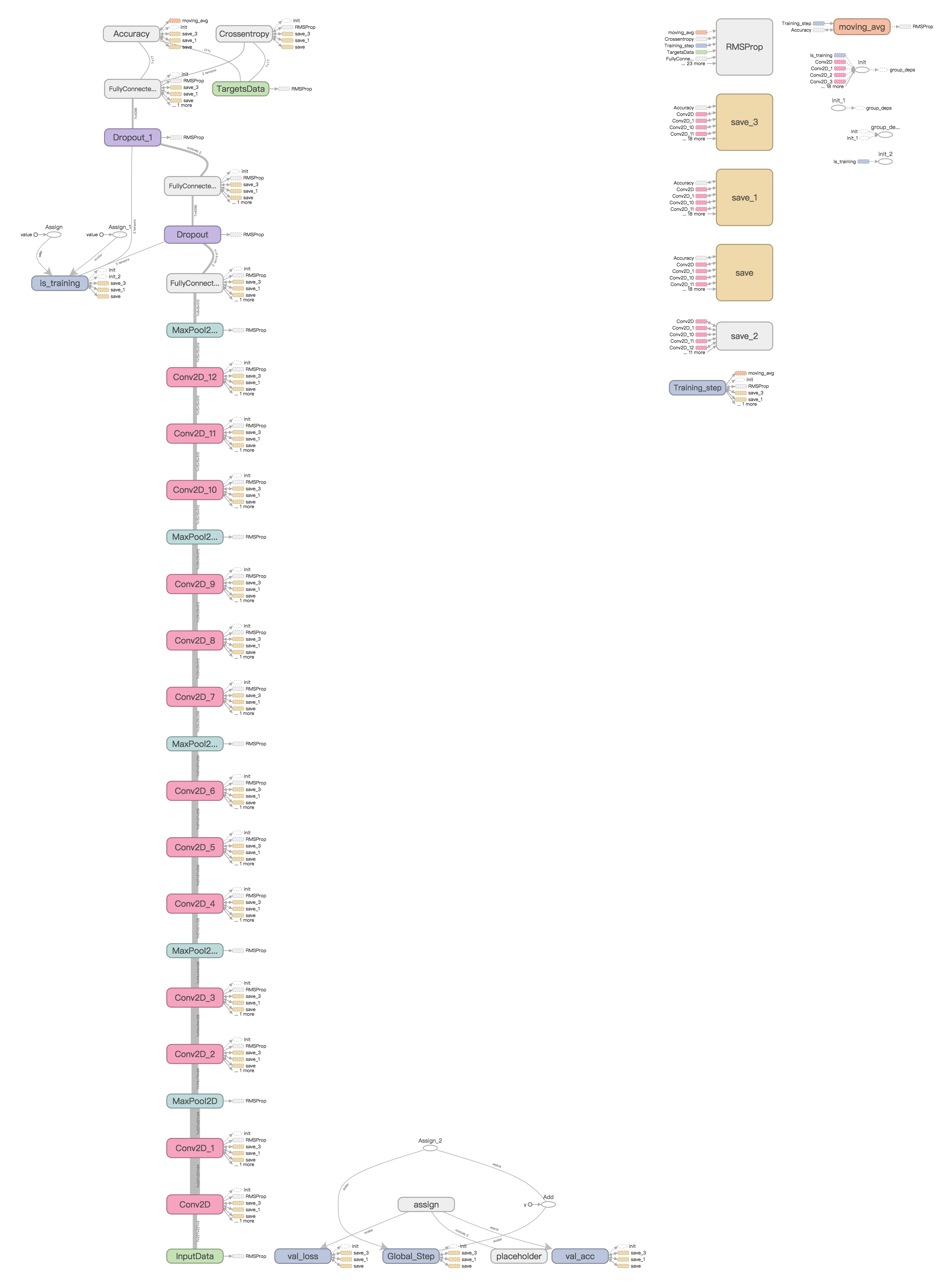# 《Web安全之深度学习实战》笔记：第二章 卷积神经网络``````import tflearn
from tflearn.layers.core import input_data, dropout, fully_connected
from tflearn.layers.conv import conv_2d, max_pool_2d
from tflearn.layers.normalization import local_response_normalization
from tflearn.layers.estimator import regression

import tflearn.datasets.mnist as mnist

import tflearn.datasets.oxflower17 as oxflower17

def cnn():
X, Y, testX, testY = mnist.load_data('c://data/MNIST/', one_hot=True)
X = X.reshape([-1, 28, 28, 1])
testX = testX.reshape([-1, 28, 28, 1])

# Building convolutional network
network = input_data(shape=[None, 28, 28, 1], name='input')
network = conv_2d(network, 32, 3, activation='relu', regularizer="L2")
network = max_pool_2d(network, 2)
network = local_response_normalization(network)
network = conv_2d(network, 64, 3, activation='relu', regularizer="L2")
network = max_pool_2d(network, 2)
network = local_response_normalization(network)
network = fully_connected(network, 128, activation='tanh')
network = dropout(network, 0.8)
network = fully_connected(network, 256, activation='tanh')
network = dropout(network, 0.8)
network = fully_connected(network, 10, activation='softmax')
loss='categorical_crossentropy', name='target')````````````def alexnet():
X, Y = oxflower17.load_data('c://data/17flowers/',one_hot=True, resize_pics=(227, 227))

# Building 'AlexNet'
network = input_data(shape=[None, 227, 227, 3])
network = conv_2d(network, 96, 11, strides=4, activation='relu')
network = max_pool_2d(network, 3, strides=2)
network = local_response_normalization(network)
network = conv_2d(network, 256, 5, activation='relu')
network = max_pool_2d(network, 3, strides=2)
network = local_response_normalization(network)
network = conv_2d(network, 384, 3, activation='relu')
network = conv_2d(network, 384, 3, activation='relu')
network = conv_2d(network, 256, 3, activation='relu')
network = max_pool_2d(network, 3, strides=2)
network = local_response_normalization(network)
network = fully_connected(network, 4096, activation='tanh')
network = dropout(network, 0.5)
network = fully_connected(network, 4096, activation='tanh')
network = dropout(network, 0.5)
network = fully_connected(network, 17, activation='softmax')
network = regression(network, optimizer='momentum',
loss='categorical_crossentropy',
learning_rate=0.001)

# Training
model = tflearn.DNN(network, checkpoint_path='model_alexnet',
max_checkpoints=1, tensorboard_verbose=2)
model.fit(X, Y, n_epoch=1000, validation_set=0.1, shuffle=True,
show_metric=True, batch_size=64, snapshot_step=200,
snapshot_epoch=False, run_id='alexnet')````````````def vggnet():
X, Y = oxflower17.load_data('c://data/17flowers/', one_hot=True,resize_pics=(227, 227))

# Building 'VGG Network'
network = input_data(shape=[None, 227, 227, 3])

network = conv_2d(network, 64, 3, activation='relu')
network = conv_2d(network, 64, 3, activation='relu')
network = max_pool_2d(network, 2, strides=2)

network = conv_2d(network, 128, 3, activation='relu')
network = conv_2d(network, 128, 3, activation='relu')
network = max_pool_2d(network, 2, strides=2)

network = conv_2d(network, 256, 3, activation='relu')
network = conv_2d(network, 256, 3, activation='relu')
network = conv_2d(network, 256, 3, activation='relu')
network = max_pool_2d(network, 2, strides=2)

network = conv_2d(network, 512, 3, activation='relu')
network = conv_2d(network, 512, 3, activation='relu')
network = conv_2d(network, 512, 3, activation='relu')
network = max_pool_2d(network, 2, strides=2)

network = conv_2d(network, 512, 3, activation='relu')
network = conv_2d(network, 512, 3, activation='relu')
network = conv_2d(network, 512, 3, activation='relu')
network = max_pool_2d(network, 2, strides=2)

network = fully_connected(network, 4096, activation='relu')
network = dropout(network, 0.5)
network = fully_connected(network, 4096, activation='relu')
network = dropout(network, 0.5)
network = fully_connected(network, 17, activation='softmax')

network = regression(network, optimizer='rmsprop',
loss='categorical_crossentropy',
learning_rate=0.0001)

# Training
model = tflearn.DNN(network, checkpoint_path='model_vgg',
max_checkpoints=1, tensorboard_verbose=0)
model.fit(X, Y, n_epoch=500, shuffle=True,
show_metric=True, batch_size=32, snapshot_step=500,
snapshot_epoch=False, run_id='vgg')``````

-- 展开阅读全文 --

Web安全—逻辑越权漏洞（BAC）
« 上一篇 03-13
Redis底层数据结构--简单动态字符串